# MATLAB sessions Laboratory 5MAT 275 Laboratory 5The Mas.docx

31 de Oct de 2022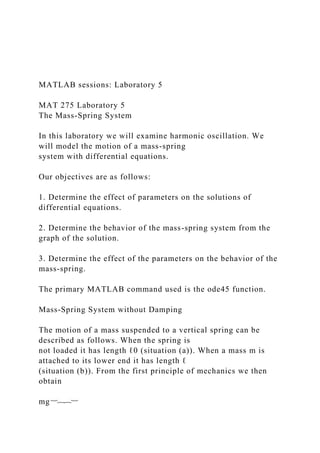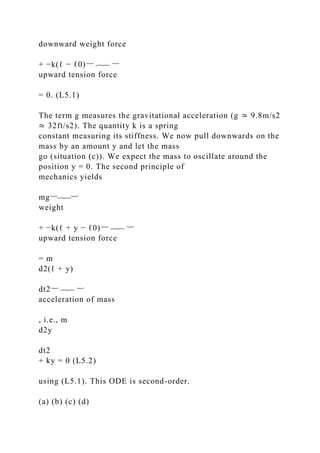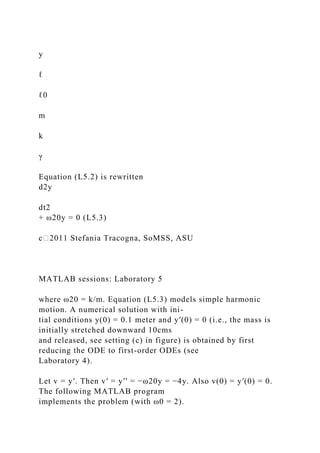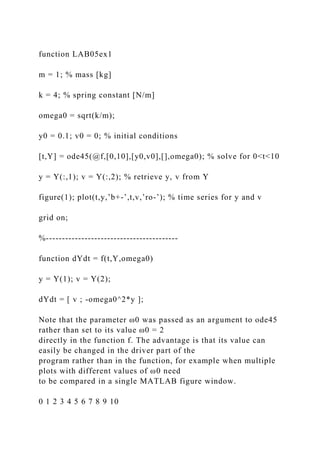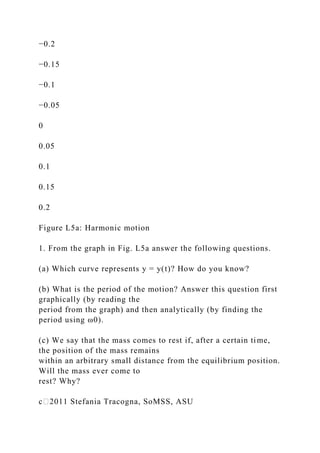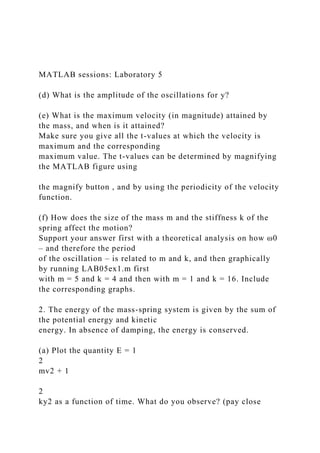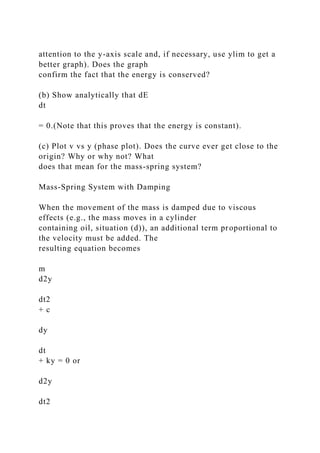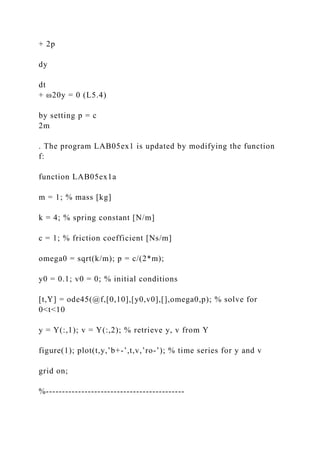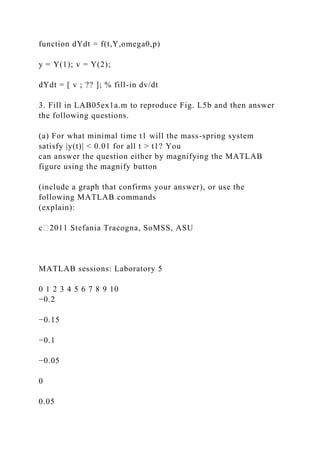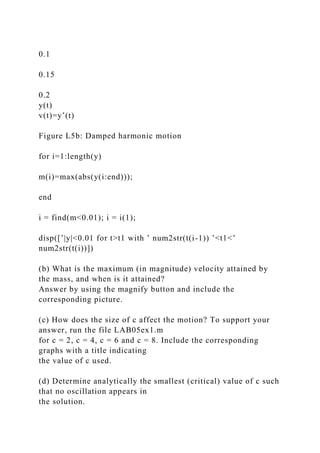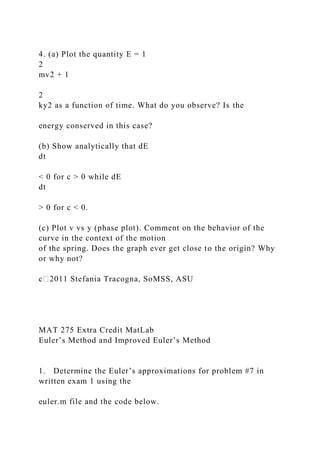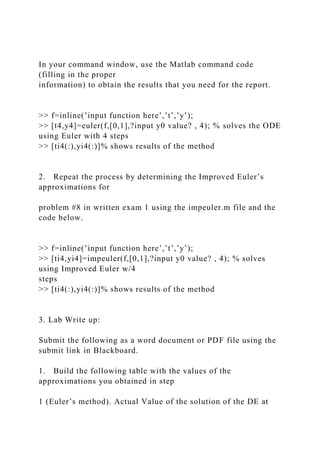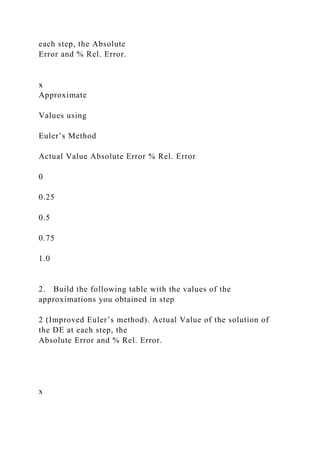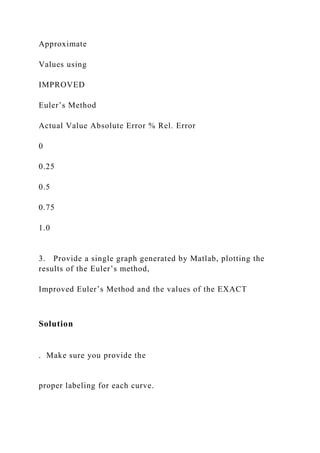Próximo SlideShare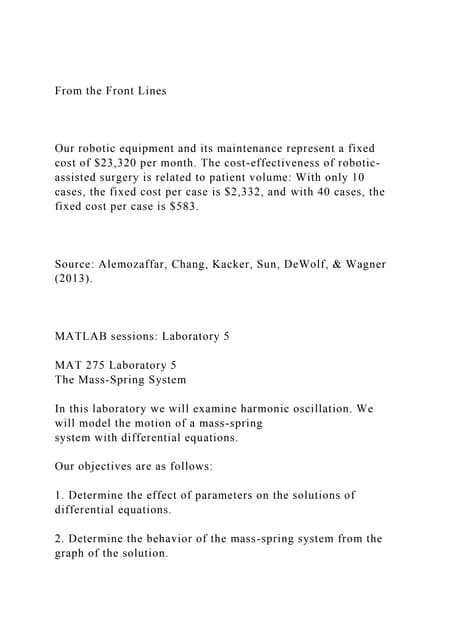From the Front LinesOur robotic equipment and its maintenanc.docx
Cargando en ... 3
1 de 15

### MATLAB sessions Laboratory 5MAT 275 Laboratory 5The Mas.docx

1. MATLAB sessions: Laboratory 5 MAT 275 Laboratory 5 The Mass-Spring System In this laboratory we will examine harmonic oscillation. We will model the motion of a mass-spring system with differential equations. Our objectives are as follows: 1. Determine the effect of parameters on the solutions of differential equations. 2. Determine the behavior of the mass-spring system from the graph of the solution. 3. Determine the effect of the parameters on the behavior of the mass-spring. The primary MATLAB command used is the ode45 function. Mass-Spring System without Damping The motion of a mass suspended to a vertical spring can be described as follows. When the spring is not loaded it has length ℓ0 (situation (a)). When a mass m is attached to its lower end it has length ℓ (situation (b)). From the first principle of mechanics we then obtain mg︸︷︷︸
2. downward weight force + −k(ℓ − ℓ0)︸ ︷︷ ︸ upward tension force = 0. (L5.1) The term g measures the gravitational acceleration (g ≃ 9.8m/s2 ≃ 32ft/s2). The quantity k is a spring constant measuring its stiffness. We now pull downwards on the mass by an amount y and let the mass go (situation (c)). We expect the mass to oscillate around the position y = 0. The second principle of mechanics yields mg︸︷︷︸ weight + −k(ℓ + y − ℓ0)︸ ︷︷ ︸ upward tension force = m d2(ℓ + y) dt2︸ ︷︷ ︸ acceleration of mass , i.e., m d2y dt2 + ky = 0 (L5.2) using (L5.1). This ODE is second-order. (a) (b) (c) (d)
3. y ℓ ℓ0 m k γ Equation (L5.2) is rewritten d2y dt2 + ω20y = 0 (L5.3) c⃝2011 Stefania Tracogna, SoMSS, ASU MATLAB sessions: Laboratory 5 where ω20 = k/m. Equation (L5.3) models simple harmonic motion. A numerical solution with ini- tial conditions y(0) = 0.1 meter and y′(0) = 0 (i.e., the mass is initially stretched downward 10cms and released, see setting (c) in figure) is obtained by first reducing the ODE to first-order ODEs (see Laboratory 4). Let v = y′. Then v′ = y′′ = −ω20y = −4y. Also v(0) = y′(0) = 0. The following MATLAB program implements the problem (with ω0 = 2).
4. function LAB05ex1 m = 1; % mass [kg] k = 4; % spring constant [N/m] omega0 = sqrt(k/m); y0 = 0.1; v0 = 0; % initial conditions [t,Y] = ode45(@f,[0,10],[y0,v0],[],omega0); % solve for 0<t<10 y = Y(:,1); v = Y(:,2); % retrieve y, v from Y figure(1); plot(t,y,’b+-’,t,v,’ro-’); % time series for y and v grid on; %----------------------------------------- function dYdt = f(t,Y,omega0) y = Y(1); v = Y(2); dYdt = [ v ; -omega0^2*y ]; Note that the parameter ω0 was passed as an argument to ode45 rather than set to its value ω0 = 2 directly in the function f. The advantage is that its value can easily be changed in the driver part of the program rather than in the function, for example when multiple plots with different values of ω0 need to be compared in a single MATLAB figure window. 0 1 2 3 4 5 6 7 8 9 10
5. −0.2 −0.15 −0.1 −0.05 0 0.05 0.1 0.15 0.2 Figure L5a: Harmonic motion 1. From the graph in Fig. L5a answer the following questions. (a) Which curve represents y = y(t)? How do you know? (b) What is the period of the motion? Answer this question first graphically (by reading the period from the graph) and then analytically (by finding the period using ω0). (c) We say that the mass comes to rest if, after a certain time, the position of the mass remains within an arbitrary small distance from the equilibrium position. Will the mass ever come to rest? Why? c⃝2011 Stefania Tracogna, SoMSS, ASU
6. MATLAB sessions: Laboratory 5 (d) What is the amplitude of the oscillations for y? (e) What is the maximum velocity (in magnitude) attained by the mass, and when is it attained? Make sure you give all the t-values at which the velocity is maximum and the corresponding maximum value. The t-values can be determined by magnifying the MATLAB figure using the magnify button , and by using the periodicity of the velocity function. (f) How does the size of the mass m and the stiffness k of the spring affect the motion? Support your answer first with a theoretical analysis on how ω0 – and therefore the period of the oscillation – is related to m and k, and then graphically by running LAB05ex1.m first with m = 5 and k = 4 and then with m = 1 and k = 16. Include the corresponding graphs. 2. The energy of the mass-spring system is given by the sum of the potential energy and kinetic energy. In absence of damping, the energy is conserved. (a) Plot the quantity E = 1 2 mv2 + 1 2 ky2 as a function of time. What do you observe? (pay close
7. attention to the y-axis scale and, if necessary, use ylim to get a better graph). Does the graph confirm the fact that the energy is conserved? (b) Show analytically that dE dt = 0.(Note that this proves that the energy is constant). (c) Plot v vs y (phase plot). Does the curve ever get close to the origin? Why or why not? What does that mean for the mass-spring system? Mass-Spring System with Damping When the movement of the mass is damped due to viscous effects (e.g., the mass moves in a cylinder containing oil, situation (d)), an additional term proportional to the velocity must be added. The resulting equation becomes m d2y dt2 + c dy dt + ky = 0 or d2y dt2
8. + 2p dy dt + ω20y = 0 (L5.4) by setting p = c 2m . The program LAB05ex1 is updated by modifying the function f: function LAB05ex1a m = 1; % mass [kg] k = 4; % spring constant [N/m] c = 1; % friction coefficient [Ns/m] omega0 = sqrt(k/m); p = c/(2*m); y0 = 0.1; v0 = 0; % initial conditions [t,Y] = ode45(@f,[0,10],[y0,v0],[],omega0,p); % solve for 0<t<10 y = Y(:,1); v = Y(:,2); % retrieve y, v from Y figure(1); plot(t,y,’b+-’,t,v,’ro-’); % time series for y and v grid on; %-------------------------------------------
9. function dYdt = f(t,Y,omega0,p) y = Y(1); v = Y(2); dYdt = [ v ; ?? ]; % fill-in dv/dt 3. Fill in LAB05ex1a.m to reproduce Fig. L5b and then answer the following questions. (a) For what minimal time t1 will the mass-spring system satisfy |y(t)| < 0.01 for all t > t1? You can answer the question either by magnifying the MATLAB figure using the magnify button (include a graph that confirms your answer), or use the following MATLAB commands (explain): c⃝2011 Stefania Tracogna, SoMSS, ASU MATLAB sessions: Laboratory 5 0 1 2 3 4 5 6 7 8 9 10 −0.2 −0.15 −0.1 −0.05 0 0.05
10. 0.1 0.15 0.2 y(t) v(t)=y’(t) Figure L5b: Damped harmonic motion for i=1:length(y) m(i)=max(abs(y(i:end))); end i = find(m<0.01); i = i(1); disp([’|y|<0.01 for t>t1 with ’ num2str(t(i-1)) ’<t1<’ num2str(t(i))]) (b) What is the maximum (in magnitude) velocity attained by the mass, and when is it attained? Answer by using the magnify button and include the corresponding picture. (c) How does the size of c affect the motion? To support your answer, run the file LAB05ex1.m for c = 2, c = 4, c = 6 and c = 8. Include the corresponding graphs with a title indicating the value of c used. (d) Determine analytically the smallest (critical) value of c such that no oscillation appears in the solution.
11. 4. (a) Plot the quantity E = 1 2 mv2 + 1 2 ky2 as a function of time. What do you observe? Is the energy conserved in this case? (b) Show analytically that dE dt < 0 for c > 0 while dE dt > 0 for c < 0. (c) Plot v vs y (phase plot). Comment on the behavior of the curve in the context of the motion of the spring. Does the graph ever get close to the origin? Why or why not? c⃝2011 Stefania Tracogna, SoMSS, ASU MAT 275 Extra Credit MatLab Euler’s Method and Improved Euler’s Method 1. Determine the Euler’s approximations for problem #7 in written exam 1 using the euler.m file and the code below.
12. In your command window, use the Matlab command code (filling in the proper information) to obtain the results that you need for the report. >> f=inline(’input function here’,’t’,’y’); >> [t4,y4]=euler(f,[0,1],?input y0 value? , 4); % solves the ODE using Euler with 4 steps >> [ti4(:),yi4(:)]% shows results of the method 2. Repeat the process by determining the Improved Euler’s approximations for problem #8 in written exam 1 using the impeuler.m file and the code below. >> f=inline(’input function here’,’t’,’y’); >> [ti4,yi4]=impeuler(f,[0,1],?input y0 value? , 4); % solves using Improved Euler w/4 steps >> [ti4(:),yi4(:)]% shows results of the method 3. Lab Write up: Submit the following as a word document or PDF file using the submit link in Blackboard. 1. Build the following table with the values of the approximations you obtained in step 1 (Euler’s method). Actual Value of the solution of the DE at
13. each step, the Absolute Error and % Rel. Error. x Approximate Values using Euler’s Method Actual Value Absolute Error % Rel. Error 0 0.25 0.5 0.75 1.0 2. Build the following table with the values of the approximations you obtained in step 2 (Improved Euler’s method). Actual Value of the solution of the DE at each step, the Absolute Error and % Rel. Error. x
14. Approximate Values using IMPROVED Euler’s Method Actual Value Absolute Error % Rel. Error 0 0.25 0.5 0.75 1.0 3. Provide a single graph generated by Matlab, plotting the results of the Euler’s method, Improved Euler’s Method and the values of the EXACT Solution . Make sure you provide the proper labeling for each curve.
15. 4. Submit a scanned copy of pages 7, 8 and 9 from your written exam 1 with the lab report. If these pages are not included no extra credit can be assigned. 5. Submit the entire document using the link in blackboard.